# How to use COUNTA function in Microsoft Excel

To count the number of cells containing data in a range in Microsoft Excel we will use COUNTA function.

COUNTA:This function is used for count the non blank or occupied cells in a range It is capable of looking thousands of cell values together at once in Microsoft Excel.

Syntax of COUNTA:-

COUNTA(value1, [value2], ...)

Now lets see the various formula types based on the arguments in this function -

Argument Type Formula Types Explanation
Cell References =COUNTA(A1, B1) Count if cells A1 and B1 are occupied
Range Reference =COUNTA(A1:A10) Count how many occupied cells are in cells A1 to A10
Column Reference =COUNTA(C:C) Count how many occupied cells are in column C
Row Reference =COUNTA(1:1) Count how many occupied cells are in row 1
Multiple Columns/Rows =COUNTA(A1:A10, C1:C10) Count how many occupied cells are in A1 to A10 and from C1 to C10
Cell and Range Names =COUNTA(Sales_2012) Count how many occupied cells are in the range named Sales_2012 *

Count if cells A1 and B1 are occupied:-

• Cell A1 contains text and cell B1 contains a numeric value.
• Write the formula in cell C1.
• =COUNTA(A1, B1), press Enter on the keyboard.
• The function will return 2, which means that both cells have data.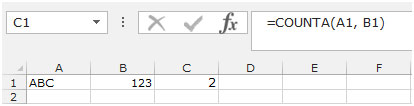Count how many occupied cells are in cells A1 to A10

• We have names in the range A1:A10 and some cells are blank.
• Write the formula in cell C1.
• =COUNTA(A1:A10), press Enter on the keyboard.
• The function will return 8 which means 8 cells are occupied.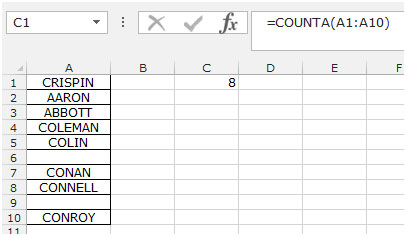Count how many occupied cells are in column A

• Column A contains names and some cells are blank.
• Write the formula in cell C1.
• =COUNTA(A:A) and press Enter on the keyboard.
• The function will return 10 which means 10 cells are occupied in the entire column.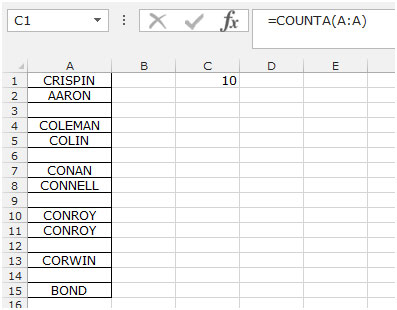Count how many cells are occupied in row 1

• We have names in the first row A1:G1 and some cells are blank.
• Write the formula in cell A3.
• =COUNTA(A1:A10) and press Enter on the keyboard.
• The function will return 5 which means 5 cells are occupied in the row.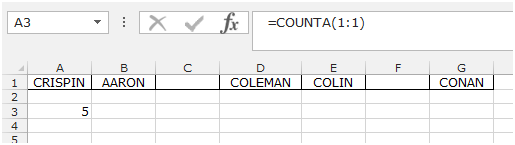Count how many cells are occupied in A1:A10 and C1:C10

Ranges A1:A10 and C1:C10 contain namesand some cells are blank. To identify how many cells are occupied follow the below given steps:-

• Write the formula in cell D1.
• =COUNTA(A1:A10, C1:C10) and press Enter on the keyboard.
• The function will return 15 which means 15 cells are occupied out of the 20 cells in both the ranges.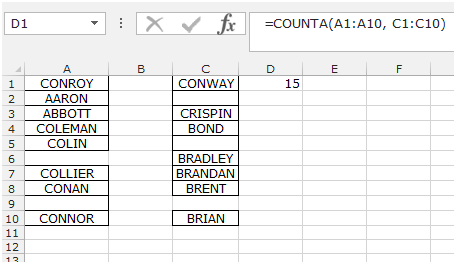Conclusion:- Thus, the COUNTA function counts information, including error values but excludes empty cells.

We would love to hear from you, do let us know how we can improve, complement or innovate our work and make it better for you. Write us at info@exceltip.com

1."Hi Joe,

If your data set is in A1:A10, then the following formula will count the number of entries greater than 1 and less than 2:

=SUM(((A1:A10)>1)*((A1:A10)<2))

{If you see the characters < in the above formula, please replace with the 'less than' sign - it is an HTML rendering issue on the board, not you or me!}

Obviously you can replace 1 and 2 with any values you like.

HTH,

Alan"

2.how do use the countif formula when I only want to select certain cells and not an entire range? I keep getting a #value symbol.

3.How do I count the number of cells that contain a number that falls between two numbers. For example, How do I count the number of cells that falls between 24 and 48 hours?

4.This is the tip I was looking for years. Now, it becomes very easy to count characters. Great tip!!!

5."If your data set is in A1:A10, then the following formula will count the number of entries greater than 1 and less than 2:

=SUM(((A1:A10)>1)*((A1:A10)<2))

{If you see the characters < in the above formula, please replace with the 'less than' sign - it is an HTML rendering issue on the board, not you or me!}

Obviously you can replace 1 and 2 with any values you like. "

6.how do use the countif formula when I only want to select certain cells and not an entire range? I keep getting a #value symbol.

7.How do I count the number of cells that contain a number that falls between two numbers. For example, How do I count the number of cells that falls between 24 and 48 hours?

Terms and Conditions of use

The applications/code on this site are distributed as is and without warranties or liability. In no event shall the owner of the copyrights, or the authors of the applications/code be liable for any loss of profit, any problems or any damage resulting from the use or evaluation of the applications/code.